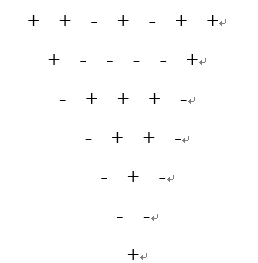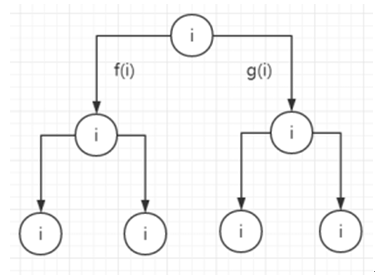﻿ 算法——回溯法

### 算法——回溯法

2021/11/17 12:49:52

• 回溯法
• 1.概述
• 2.三个概念
• 3.解题步骤
• 一：符号三角形问题
• 1.问题描述
• 2.解题思路
• 3.程序代码
• 二：整数变换问题
• 1.问题描述
• 2.程序代码
• 三：子集和问题
• 1.问题描述
• 2.程序代码
• 四：工作分配问题
• 1.问题描述
• 2.程序代码

# 回溯法

## 2.三个概念

**约束函数：**约束函数是根据题意定出的。通过描述合法解的一般特征用于去除不合法的解，从而避免继续搜索出这个不合法解的剩余部分。因此，约束函数是对于任何状态空间树上的节点都有效、等价的。
**状态空间树：**一个问题的解可以表示成解向量X = (x1, x2, …, xn)，X中每个分量xi所有取值的组合构成问题的解向量空间，简称解空间或者解空间树，又称为状态空间树，是一个对所有解的图形描述。树上的每个子节点的解都只有一个部分与父节点不同。
**扩展节点、活结点、死结点：**所谓扩展节点，就是当前正在求出它的子节点的节点，在DFS中，只允许有一个扩展节点。活结点就是通过与约束函数的对照，节点本身和其父节点均满足约束函数要求的节点；死结点反之。由此很容易知道死结点是不必求出其子节点的（没有意义）。

## 3.解题步骤

(1)描述解的形式，定义一个解空间，它包含问题的所有解，这一步主要明确问题的解空间树。
(2)确定结点的扩展搜索规则。
(3)构造约束函数（用于杀死节点）。然后就要通过DFS思想完成回溯，DFS可以采用递归回溯或者非递归（迭代）回溯。 确定问题的解空间 -->

# 一：符号三角形问题

## 1.问题描述1. 分别输出n的值为1----20时，对应的符号三角形个数，如没有满足条件的符号三角形，则输出0
2. 输入一个整数n，输出对应的符号三角形的个数，并依次显示出所有的符号三角形。

## 2.解题思路

（1）解题思路：
1、不断改变第一行的每个符号，搜索符合条件的解，可以使用递归回溯。

++为+即0^0=0, --为+即1^1=0, ±为-即0^1=1, -+为-即1^0=1
2、因为两种符号个数相同，可以对题解树剪枝

（2）算法设计

## 3.程序代码

``````public  class Triangles{
static int n, //第一行符号的个数
count, //n*(n+1)/2
half; //当前"+"的个数
static int [][] p; //符号三角形矩阵
static long sum; // 已找到的符号三角形数

public static long compute(int nn)
{
n=nn;
count=0;
sum=0;
half=n*(n+1)/2;
if (half%2==1) return 0;
half=half/2;
p=new int[n+1][n+1];
for(int i=0;i<=n;i++)
for(int j=0; j<=n;j++)
p[i][j]=0;
backtrack(1);
return sum;
}

public static void backtrack (int t)
{
if((count>half)||(t*(t-1)/2-count>half))
{
return;  //剪去不满足约束的子树
}
if(t>n)
{
sum++; //找到一个满足要求的三角形
/*
可在此处加入代码，输出当前解对应的符号三角形
*/
}
else
for(int i=0;i<2;i++)
{//子树
p[t]=i;
count+=i;
for(int j=2;j<=t;j++) //该子树形成的三角形
{
p[j][t-j+1]=p[j-1][t-j+1]^p[j-1][t-j+2];
count+=p[j][t-j+1];
}
backtrack(t+1);
for(int j=2;j<=t;j++) //回溯恢复
count-=p[j][t-j+1];
count-=i;
}
}

public static void main(String[] args) {
int n=4; long result;
result=compute(n);
System.out.println(String.format("result=%d",result));
}
}

``````
``````package suanfa;
public class suanfa1 {

static int n;//第一行的符号个数
static int half;//n*(n+1)/4
static int count;//当前“+”或者“-”的个数
static int[][] p;//符号三角形矩阵
static long sum;//已找到的符号三角形的个数

public static float Compute(int nn) {
n = nn;
count = 0;
sum = 0;
half = (n*(n+1))>>1;
if((half>>1)<<1 != half) {
return 0;
}
half = half>>1;
p = new int[n+1][n+1];
backtrack(1);

return sum;
}

/**
* 算法1
* @param t
*/

public static void backtrack01(int t) {
if(count>half || (t*(t-1)/2-count > half)) {//对题解树的剪枝
return;
}
if(t>n) {
sum++;//符号三角形的总数目+1
}
else {
//每个位置都有两种情况0,1
for(int i = 0;i<2;i++) {
p[t] = i;
count += i;//对"-"个数进行技术，为了进行剪枝操作

//接下来绘制其余的n-1行
for(int j = 2;j<=t;j++) {
//通过异或的方式求其余行数的放置方式
p[j][t-j+1] = p[j-1][t-j+1]^p[j-1][t-j+2];
count += p[j][t-j+1];
}
backtrack01(t+1);

//恢复现场
for(int j = 2;j<=t;j++) {
count -= p[j][t-j+1];
}
count -= i;
}
}
}

public static void backtrack(int t) {
if((count>half)||((t*(t-1)/2-count > half)) ){//对题解树的剪枝
return;
}
if(t>n) {
sum++;
//打印符号三角形
for(int i =1;i<=n;i++) {
for(int k = 1;k<i;k++) {
System.out.print(" ");
}
for(int j =1;j<=n;j++) {
if(p[i][j] == 0 && j<=n-i+1) {
System.out.print("+" + " ");
}
else if(p[i][j] == 1 && j<=n-i+1) {
System.out.print("-" + " ");
}
else {
System.out.print("  ");
}
}
System.out.println();
}
System.out.println();
}
else {
//每个位置都有两种情况0,1
for(int i =0;i<2;i++) {
p[t] = i;
count += i;//计算“-”的个数

//接下来绘制其余的n-1行
for(int j = 2;j<=t;j++) {
//通过异或的方式求其余行数的放置方式
p[j][t-j+1] = p[j-1][t-j+1]^p[j-1][t-j+2];
count += p[j][t-j+1];

}
backtrack(t+1);

//恢复现场
for(int j =2;j<=t;j++) {
count -= p[j][t-j+1];
}
count -= i;

}
}
}

public static void main(String[] args) {
// TODO Auto-generated method stub
float SUM = Compute(4);
System.out.print("总数： " + SUM);
}

}

``````

# 二：整数变换问题

## 1.问题描述

n>m,执行g,n=[9/2]=4
n<m,执行f,n=34=12
n>m,执行g,n=[12/2]=6
n>m,执行f,n=[6/2]=3
n<m,执行g,n=3
3=9
n>m,执行f,n=[9/2]=4## 2.程序代码

``````package suanfa;
import java.util.Scanner;
public class suanfa1 {
static int m,n1; //n1方便后面的输出形式
static int tempcount,bestcount;//当前变换次数，最佳变换次数
static int[] temp1=new int;
static int[] temp2=new int;
public static void main(String args[]){
Scanner input=new Scanner(System.in);
System.out.println("请输入需变换的整数");
int n=input.nextInt();
n1=n;
System.out.println("请输入需变换后的整数");
m=input.nextInt();
tempcount=0;
bestcount=100;
test(n);
}

//实现方法
public static void test(int n){
if(tempcount>99){  //最好的变换次数已经大于某个特定的值,是无解情况(隐式约束)
System.out.println("无解");
}
else{
if(n==m){          //有解情况
if(tempcount<bestcount)     //
bestcount=tempcount;
System.out.println("最佳变换次数:"+bestcount);
System.out.printf("%d=",m);
for(int i=bestcount;i>=1;i--){
if(temp2[i]==2)
System.out.print("f");
if(temp2[i]==1)
System.out.print("g");
}
System.out.printf("(%d)",n1);
}
else if(n>m){   //n>m走这个分支 ,起到剪枝作业
n=g(n);
tempcount++;
temp2[tempcount]=1;
test(n);
}
else {      //n<m走这个分支
n=f(n);
tempcount++;
temp2[tempcount]=2;
test(n);
}
}
}
//f(i)=3*i
public static int f(int n){
n=3*n;
return n;
}
//g(i)=i/2
public static int g(int n){
n=n/2;
return n;
}
}

``````

# 三：子集和问题

## 2.程序代码

``````package suanfa;
import java.util.Scanner;
public class suanfa2 {
public static void main(String[] args) {
SubSet subSet=new SubSet();
Scanner scanner = new Scanner(System.in);
System.out.println("输入长度和所求子集总和");
int len,m;
len=scanner.nextInt();
m=scanner.nextInt();
int[] nums=new int[len];//初始数组
int[] sets=new int[len];//子集数组
System.out.println("输入初始数组");
for(int i=0;i<len;i++)
nums[i]=scanner.nextInt();
subSet.setNums(nums);
subSet.setSets(sets);
subSet.setTotal(m);
subSet.setCount(0);
subSet.setErrStr("No Solution");
subSet.backTrack(0);
if(subSet.getCount()==0){
System.out.println(subSet.getErrStr());
}
}
}

``````

subSet.class

``````package suanfa;
import java.util.Arrays;
public class SubSet{
private int[] nums;//初始数组
private int[] sets;//子集数组
private int total;//和
private int count;//存在子集数
private String errStr;//没有子集的时候输入的语句

public void setErrStr(String errStr) {
this.errStr = errStr;
}
public void setNums(int[] nums) {
this.nums = nums;
}
public void setSets(int[] sets) {
this.sets = sets;
}
public void setTotal(int total) {
this.total = total;
}
public void setCount(int count) {
this.count = count;
}
public int[] getNums() {
return nums;
}
public int[] getSets() {
return sets;
}
public int getTotal() {
}
public int getCount() {
return count;
}
public String getErrStr() {
return errStr;
}
public void backTrack(int idx){
if(total==0){
System.out.println(Arrays.toString(sets));
count++;
return;
}
if(idx==nums.length||total<0)
return;
else{
for(int i=idx;i<nums.length;i++){
if(nums[i]<=total){
sets[i]=nums[i];
total-=nums[i];
backTrack(i+1);
sets[i]=0;
total+=nums[i];
}
}
}
}
}

``````

``````4  31
13 24 11 7
``````

``````[13, 0, 11 , 7]
[0, 24, 0 , 7]
``````

``````5  235
123 55 9 4 11
``````

``````No Solution
``````

# 四：工作分配问题

3
10 2 3
2 3 4
3 4 5

9

## 2.程序代码

``````package suanfa;
import java.util.Scanner;
public class suanfa3 {
public static int sum = 0,n;
public static int [] [] num ;
public static boolean []  bool;
public static int min = Integer.MAX_VALUE;
public static void main(String[] args) {
Scanner sc =new Scanner(System.in);
n = sc.nextInt();
num = new int [n+1][n+1];
bool = new boolean [n+1];
for (int i = 1; i <=n; i++) {
for (int j = 1; j <=n; j++) {
num[i][j]=sc.nextInt();
}
}
f(1);
System.out.println(min);
}
public static void f(int a){
if(a==n+1){
if(sum<min){
min=sum;
}
return;
}
for (int i = 1; i <=n; i++) {
if(!bool[i]){
sum+=num[a][i];
bool[i]=true;
f(a+1);
sum-=num[a][i];
bool[i]=false;
}
}
}
}

``````